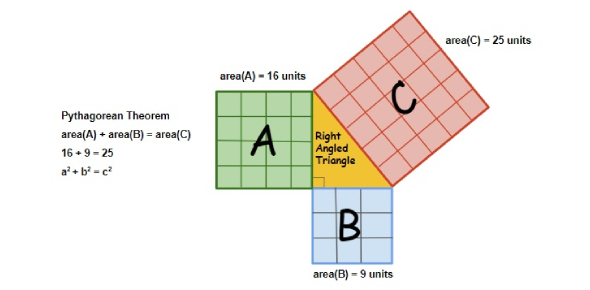Why is the Pythagorean theorem a special case of the law of the - ProProfs DiscussTopicsMoreProductsMore+ Ask Question

# Why is the Pythagorean theorem a special case of the law of the cosines?

Asked by K. Tanaka, Last updated: Feb 26, 2020

###Request 0FollowShareAnswer AnonymouslyAnswer LaterCopy Link#### John F. connor

Traveler, Avid Reader, Free as a bird

John F. connor, Content Marketing executive, MA, Minsk,PolandThe law of the cosines involves a triangle where it relates to the angles and not the sides like it does with the Pythagorean Theorem. However, there is also a formula for the law of cosines and it is similar to the formula for the Pythagorean Theorem. It states C squared is equal to A squared plus B squared minus two A times B times the cosine times the measurement of the angle that is between the lines of A and B.The angle that is labeled is actually situated across from the line with the same letter. The Pythagorean Theorem is a special case of the law of cosines because the formulas are so similar, but the law of cosines can be used for any triangle.Search for Google imagesSelect a recommended image
Upload from your computerCancelSearch for Google imagesSelect a recommended image
Upload from your computerCancelSearch for Google imagesSelect a recommended image
Upload from your computerCancel